DE / EN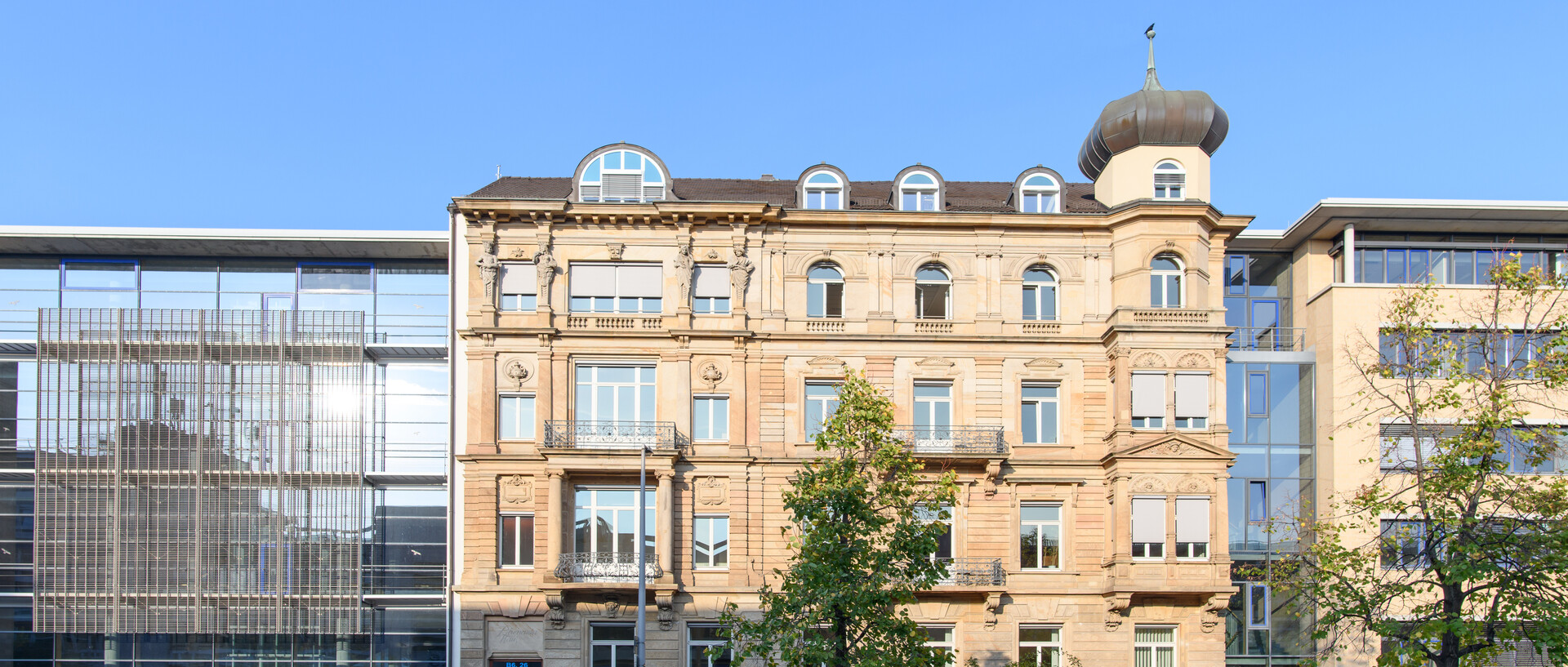# Research at the Institute of Mathematics

The research of our institute concentrates on the mathematical modelling and analysis of complex systems, from pure Mathematics to Operations Research. Typical applications can be found in natural sciences, economics, business and industry. On this page, you can find an overview of the individual research groups at the institute and a brief description of their research.

## Chairs at the institute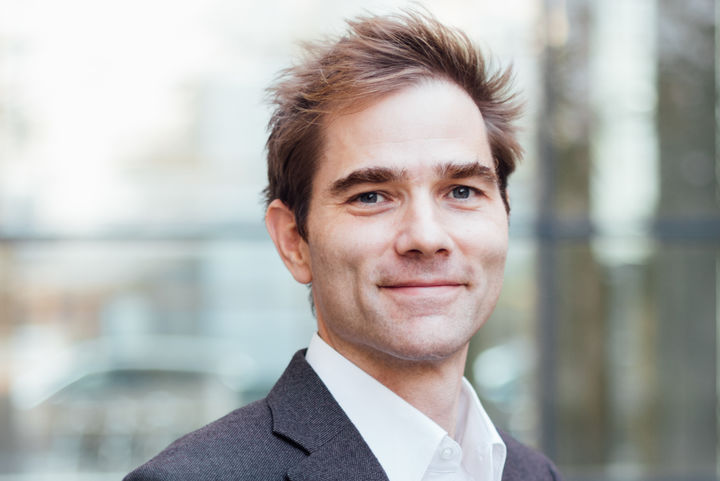##### Chair of Stochastic Numerics

Prof. Dr. Andreas Neuenkirch

The chair’s main research focus is on stochastic numerics. We are currently exploring numerical methods for stochastic differential equations relating to the (fractional) Brownian motion. Many of these equations can be applied in the natural sciences, mathematical finance or other areas.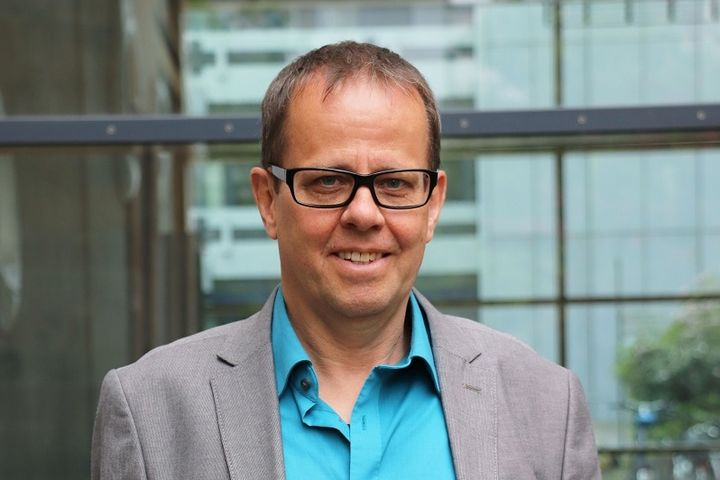##### Chair of Geometric Analysis

Prof. Dr. Martin Schmidt

The chair’s main research focus is on geometric analysis. We are currently examining spectral curves of periodic differential operators and the geometric analysis of surfaces in three-dimensional spaces, with special emphasis on surfaces with constant mean curvature.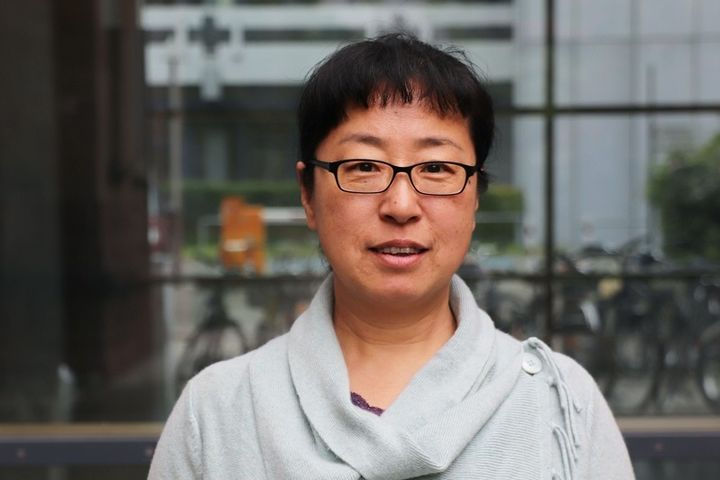##### Chair of Applied Analysis

Prof. boshi. Li Chen

The chair’s main research focus is on applied analysis, with a special focus on partial differential equations and their applications. Our current topics of research include kinetic and diffusion equations, singular limits in partial differential equations, and the derivation of effective single-particle equations for multi-particle systems.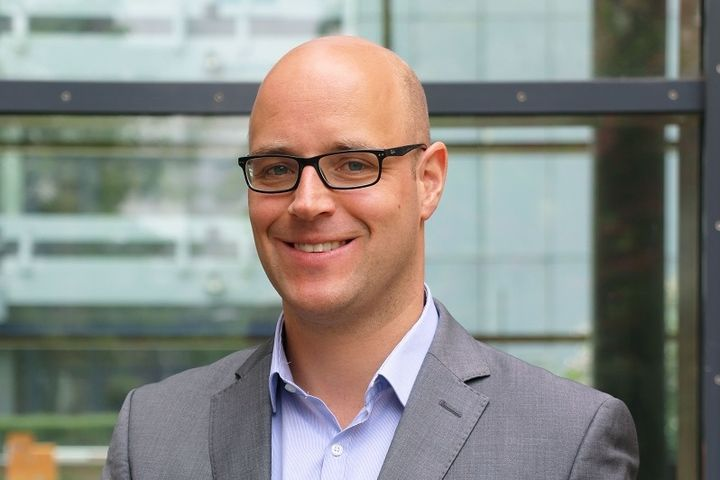##### Chair of Stochastics

Prof. Dr. Leif Döring

The chair’s main research focus is on theoretical and statistical questions for stochastic processes, such as stochastic differential equations (with jumps), Lévy processes, branching processes, and self-similar Markov processes. Processes of this kind have various applications in mathematical finance and insurance mathematics as well as other fields.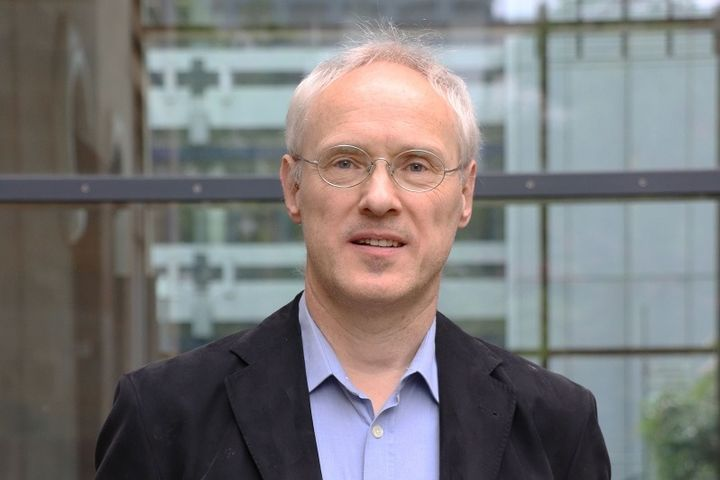##### Chair of Algebraic Geometry

Prof. Dr. Claus Hertling

The chair’s main research focus is on algebraic geometry. We explore singularities in analytic and algebraic geometry. Other topics include Frobenius manifolds, Painlevé equations, meromorphic relations and differential equations, moduli spaces, and period mappings.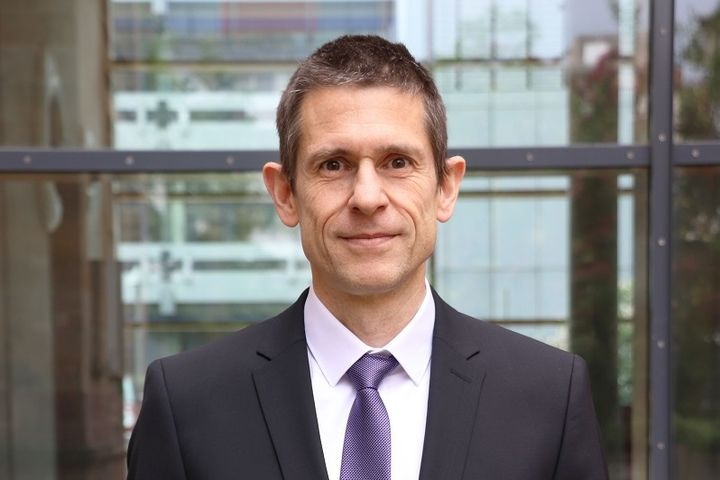##### Chair of Applied Stochastics

Prof. Dr. Martin Schlather

The chair’s main research focus is on computational statistics and data science, extreme value theory, geostatistics, and stochastic geometry. The topics we examine have multiple applications, for instance in ecology, finance, forestry, genetics, marketing, and meteorology.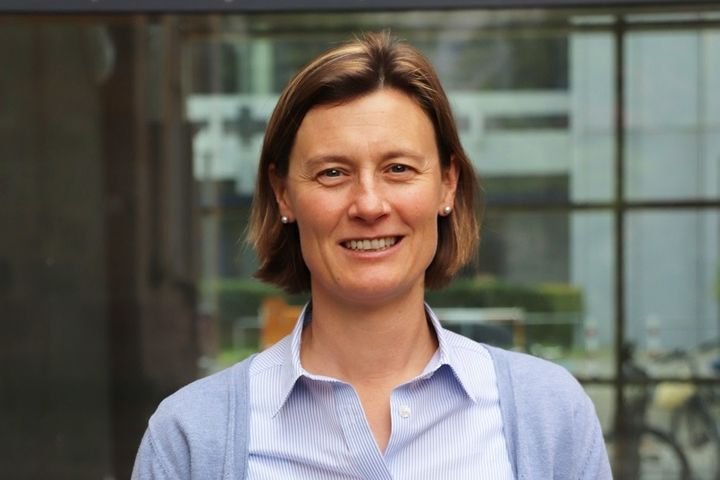##### Chair of Scientific Computing

Prof. Dr. Simone Göttlich

The chair’s main research focus is on mathematical modeling, numerical simulation, and optimizing dynamic processes. The topics we examine have multiple applications, for instance in manufacturing systems, traffic flow models, and energy networks.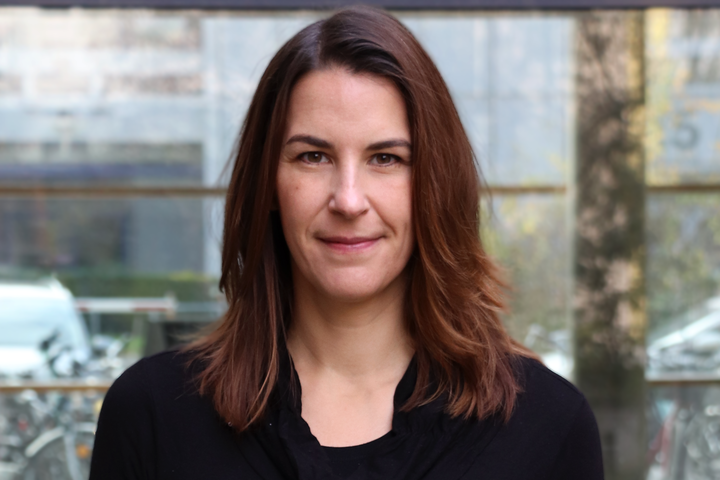##### Chair of Mathematical Optimization

Prof. Dr. Claudia Schillings

The chair’s main research focus is on applied and numeric mathematics, especially optimization, inverse problems and uncertainty quantification. Areas of application include engineering, physical and biological systems, biochemical networks, and finance.##### Chair of Mathematical Physics

Prof. Dr. Daniel Roggenkamp

The chair’s main research focus is on conformal and topological quantum field theory, particularly defects, string theory, applied topology, persistent homology, and topological data analysis.

##### Chair of Pure Mathematics

Apl.-Prof. Dr. Wolfgang K. Seiler

The chair’s main research focus is on moduli and deformations of algebraic surfaces, Galois superpositions of affine lines, and applications of algebraic geometry, with a special focus on constructive methods for solving nonlinear systems of equations, and applications in cryptology.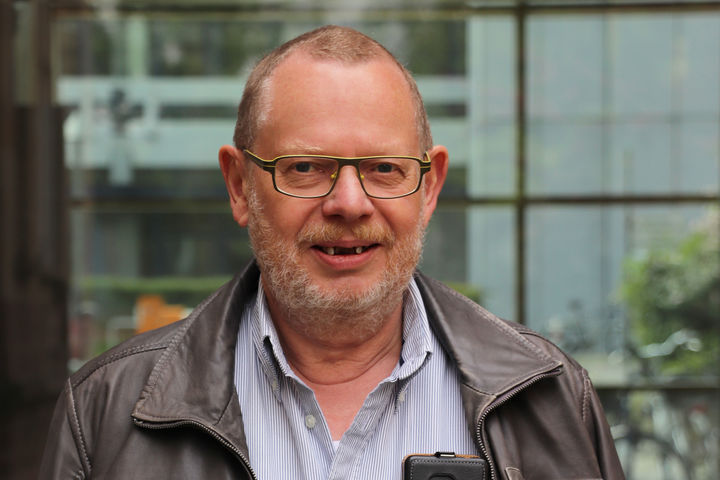##### Distinguished Retired Professor for Insurance Mathematics

Prof. Dr. Klaus D. Schmidt

Research interests include non-life actuarial mathematics (especially risk models, tariffication, loss reserving and reinsurance) and related areas of probability theory and statistics (with emphasis on linear models, copulas and measures of concordance).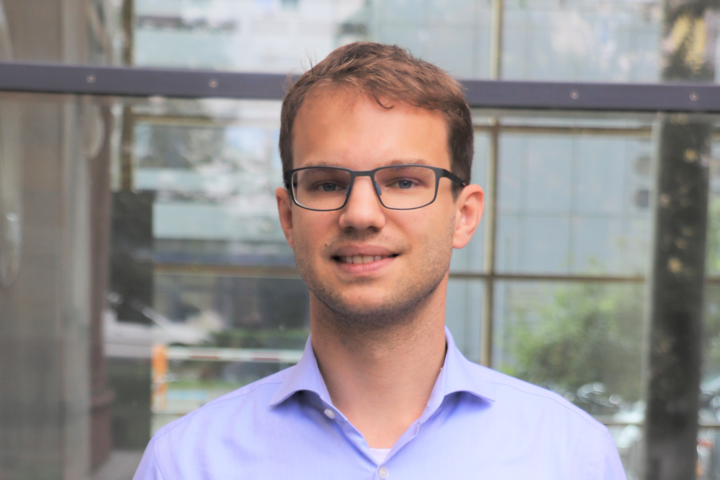##### Junior Professor for Mathematical Finance

Prof. Dr. David Prömel

Our main research focus is on mathematical finance and stochastic analysis. Current areas of research include in particular model-free mathematical finance, pathwise stochastic calculus (e.g. rough paths and regularity structures) and stochastic (partial) differential equations, which serve as a basis for mathematical modeling in different areas.